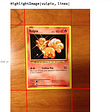# A. Promise

1. 掌握 SRCNN Paper 的重點。

# 0. Abstract (綱要)

• 此團隊針對 Super Resolution 提出了一套深度學習的方法。→ 也就是 SRCNN
• 這個方法是一個 CNN-based 的 Model，Input 是一張 low-resolution 的照片，而 Output 是一張 high-resolution 的照片。
• 可以把傳統的 sparse-coding-based SR 方法也視為 deep CNN 的方法。
• 此為相當輕量的模型，且保持高品質的輸出。

# 1. Introduction (介紹)

## Sparse-coding-based method 有四步驟

1. Overlapping patches are densely cropped from the input image and pre-processed (e.g., subtracting mean and normalization)
2. These patches are then encoded by a low-resolution dictionary.
3. The sparse coefficients are passed into a high-resolution dictionary for reconstructing high-resolution patches.
4. The overlapping reconstructed patches are aggregated (e.g., by weighted averaging) to produce the final output.

## 以下為作者等人提到的 SRCNN 的優點

• Its structure is intentionally designed with simplicity in mind, and yet provides superior accuracy compared with state-of-the-art example-based methods.
• With moderate number of filters and layers, our method achieves fast speed for practical on-line usage even on a CPU.
• Experiments show that the restoration quality of the network can be further improved when (i) larger and more diverse datasets are available and/ or (ii) a larger and deeper model is used.

# 3. Convolutional Neural Networks For Super-Resolution (CNN for SR)

## 3.1.1 Patch extraction and representation

• This operation extracts (overlapping) patches from the low-resolution image Y and represents each patch as a high-dimensional vector.
• These vectors comprise a set of feature maps, of which the number equals to the dimensionality of the vectors.
• W1 and B1 represents the filters and biases respectively, and * denotes the convolutional operation.
• W1 corresponds to n1 filters of support c x f1 x f1, where c is the number of channels in the image, f1 is the spatial size of a filter.
• Intuitively, W1 applies n1 convolutions on the image, and each convolution has a kernel size c x f1 x f1.
• B1 is an n1-dimensional vector.
• Apply ReLU.

## 3.1.2 Non-linear mapping

• This operation nonlinearly maps each high-dimensional vector onto another high-dimensional vector.
• Each mapped vector is conceptually the representation of a high-resolution patch.
• These vectors comprise another set of feature maps.
• W2 contains n2 filters of size n1 x f2 x f2, and B2 is n2-dimensional.

## 3.1.3 Reconstruction

• This operations aggregates the above high-resolution patch-wise representations to generate the final high-resolution image.
• This image is expected to be similar to the ground truth X.
• W3 corresponds to c filters of a size n2 x f3 x f3, and B3 is a c-dimensional vector.

## 3.2 Relationship to Sparse-Coding-Based Methods

• In non-linear mapping operator, its spatial support is 1 x 1.
• And, it’s an iterative algorithm, not feed-forward algorithm.
(也就是說，像是用兩個 loop 去對每個 pixel 做運算。)
• On the contrary, our non-linear operator is fully feed-forward and can be computed efficiently.

## 3.3 Training

• Network Parameters Θ ={W1, W2, W3, B1, B2, B3}
• The filter weights of each layer are initialized by drawing randomly form a Gaussian distribution with zero mean and standard deviation 0.001 (and 0 for biases.)
• Use the loss between the reconstructed images F(Y;Θ) and the corresponding ground truth high-resolution images X.
• Use Mean Square Error (MSE) as the loss function.
• n is the number of the training examples.
• The loss is minimized using stochastic gradient descent with standard backpropagation.
• The learning rate is 1e-4 for the first two layers, and 1e-5 for the last layer. They empirically find that a smaller learning rate in the last layer is important for the network to converge.

# 4. Experiments (實驗)

• We first investigate Impact of using different datasets on the model performance.
• Next, they examine the filters learned by their approach.
• Then, We explore different architecture designs of the networks. And, study the relationship between super-resolution performance and factors like depth, number of filters, and filter sizes.

## 4.2 Learned Filters for Super-Resolution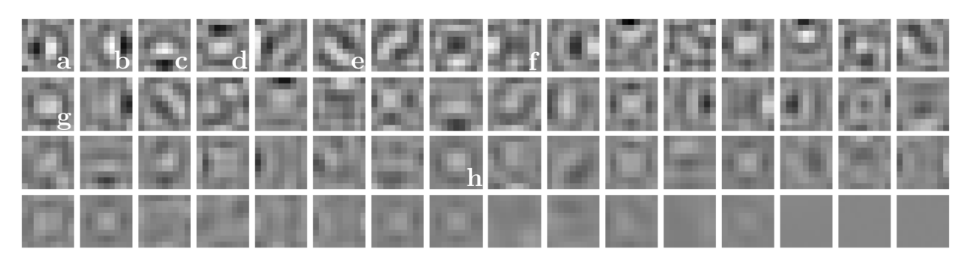These are filters trained on the ImageNet by an upscaling factor 3.

## 4.3.1 Filter number

• In general, the performance would improve if we increase the network width, i.e. adding more filters , at the cost of running time.
• We conduct two experiments: (i) one is with a larger network with n1 = 128 and n2 = 64. (ii) The other is with a smaller network with n1 = 32 and n2 = 16.

## 4.3.2 Filter size

• In this section, they examine the network sensitivity to different filter sizes.
• We fix the filter size f1 = 9. f3 = 5, and enlarge the filter size of the second layer to be (i) f2 = 1 (9–1–5). (ii) f2 = 3 (9–3–5). (iii) f2 = 5 (9–5–5).
• Convergence curves in the above figure show that using a larger filter size could significantly improve the performance.
• The results suggest that utilizing neighborhood information in the mapping stage is beneficial.

## 4.3.3 Number of layers

• We try to deeper structures by adding another non-linear mapping layer.
• We conduct 3 controlled experiments, i.e., (i) 9–1–1–5, (ii) 9–3–1–5, (iii) 9–5–1–5.

## 4.4 Comparisons to State-of-the-Arts

• SC: sparse coding-based method of Yang et al. 
• NE+LLE: neighbour embedding + locally linear embedding method 
• ANR: Anchored Neighbourhood Regression method 
• A+L: Adjusted Anchored Neighbourhood Regression method 
• KK: the method described in 

# 5. Conclusions (結論)

• We have presented a novel deep learning approach for single image super-resolution (SR)
• We show that conventional sparse-coding-based SR methods can be reformulated into a deep convolutional neural network. → Section 3.2
• With a lightweight structure, the SRCNN has achieved superior performance than the state-of-the-art methods. → Section 4.4
• We conjecture that additional performance can e further gained y exploring more filters and different training strategies.
• The proposed structure, with its advantages of simplicity and robustness, could e applied to other low-level vision problems, such as image de￾
blurring or simultaneous SR+denoising.
• One could also investigate a network to cope with different upscaling factors.

# Online Resources: 本篇文章使用到的網路資源

1. [Link] Image Super-Resolution Using Deep Convolutional Networks

# 2. Related Work (前人文獻)

--

--

--

## More from AI.FREE

Love podcasts or audiobooks? Learn on the go with our new app.

## Building PayPal-BERT using Transfer Learning and Self-Supervised Learning Techniques## GPT-3 An Overview## A Spark Attack## Exploring Classifiers with Python Scikit-learn — Iris Dataset## Hyper-Parameter Tuning!!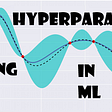## Graph Neural Networks and Generalizable Models in Neuroscience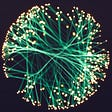## OCR on Region of Interest (ROI) in image using OpenCV and Tesseract## Introduction to Logistic Regression and its Mathematical Implementation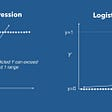## Remove shadows and uneven lighting from photographs of writing on paper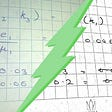## Canny edge detection in OpenCV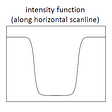## How to detect the face of live video Streaming and blur the face Using Opencv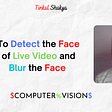## Building a Pokémon Card Detector using Mathematica